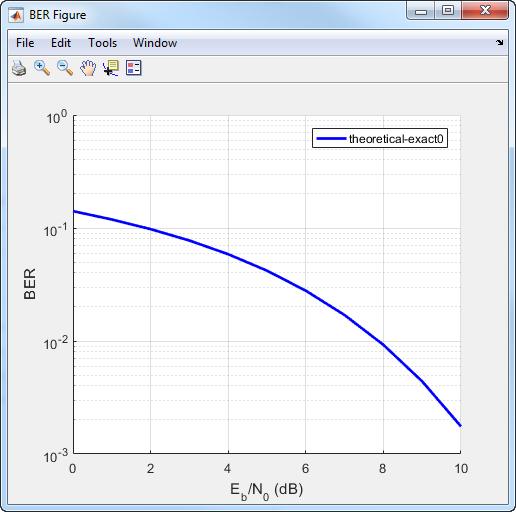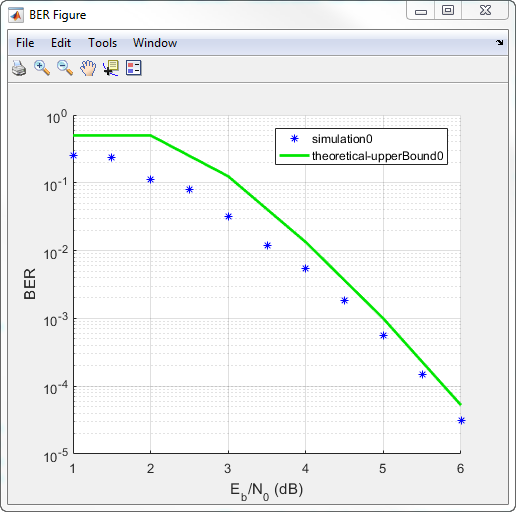Documentation

### This is machine translation

Mouseover text to see original. Click the button below to return to the English version of the page.

# BER Analyzer

Analyze bit error rate (BER) performance of communications systems

## Description

The BER Analyzer app calculates BER as a function of the energy per bit to noise power spectral density ratio (Eb/N0). Using this app, you can:

• Plot theoretical BER vs. Eb/N0 estimates and upper bounds.

• Plot BER vs. Eb/N0 using the semianalytic technique. The semianalytic technique estimates BER performance by using a combination of simulation and analysis. Use this technique when the system error rate is small, for example, < 10–6.

• Estimate BER performance by using MATLAB® functions or Simulink® models.## Open the BER Analyzer App

• MATLAB Toolstrip: On the Apps tab, under Signal Processing and Communications, click the app icon.

• MATLAB command prompt: Enter `bertool`.

## Examples

expand all

Generate a theoretical estimate of BER performance for a 16-QAM link in AWGN.

Open the BER Analysis app.

`bertool`

Specify the Eb/N0 range as `0:10`.

Set Modulation type to `QAM` and Modulation order to `16`.Plot the BER curve by clicking .Use the semianalytic technique to plot the BER for a QPSK link having rectangular pulses.

Open the BER Analysis app.

`bertool`

On the Semianalytic tab, set these parameters:

• Set the Modulation order to `4`.

• Set the Samples per symbol parameter to `8`.

• Set the Transmitted signal and Received signal parameters to `rectpulse(pskmod([0:3 0],4),8)`. To use the semianalytic technique, the number of symbols must exceed ML, where M is the modulation order and L is the impulse response length. The impulse response is 1, so a minimum of five symbols is required.

• Specify the Numerator as `ones(8,1)/8`. These coefficients specify an ideal integrator having eight samples per symbol.Plot the BER vs. Eb/N0 curve by clicking Plot.Simulate BER using a custom MATLAB function.

Open the BER Analysis app.

`bertool`

On the Monte Carlo tab, specify Eb/N0 range as `1:.5:6`.

To plot estimated BER values, run the simulation by clicking Run.

On the Theoretical tab, specify Eb/N0 range as `1:6` and set Modulation order to `4`.

Enable convolutional coding by selecting the check box.

Plot the upper bound of the BER curve by clicking Plot.## Parameters

expand all

#### Theoretical

Specifies the range of Eb/N0 values, in dB, over which the BER is evaluated. The values in the range vector must be real.

Example: `5:10`

Specifies the type of channel over which the BER is evaluated. The `Rayleigh` and `Rician` options correspond to flat fading channels.

Specifies the modulation type of the communication link.

Specifies the modulation order of the communication link.

Specifies if the input data sequence is differentially encoded.

Specifies if `Coherent` or `Noncoherent` demodulation is used. This parameter is available only when the Modulation type is `FSK` or `MSK`.

Specifies the type of channel coding used to estimate the theoretical BER.

Specifies the synchronization error in the demodulation process. This parameter is available only when the Modulation type is `PSK` and the Modulation order is `2`.

• When Synchronization is ```Normalized timing error```, specify the normalized error as a real number from `0` to `0.5`.

• When Synchronization is ```RMS phase noise level```, specify the RMS phase noise as a nonnegative real number.

Specify the method used to decode the received data. This parameter is available when either of these conditions exist:

• Channel coding is set to Convolutional

• Channel coding is set to Block and Coding Type is `General`

Specify the convolutional code trellis as a structure variable. You can generate this structure by using the `poly2trellis` function. The parameter is available only when the Channel coding parameter is `Convolutional`.

Specify the block code used in the BER evaluation.

Specify the codeword length as a positive integer.

Specify the message length as a positive integer such that K is less than N.

Specify the minimum distance of the (N,K) block code as a positive integer. This parameter is available when the Coding type is `General`.

#### Semianalytic

Specify the number of samples per symbol as a positive integer.

Specify the transmit sequence as a real or complex column vector.

Data Types: `double`
Complex Number Support: Yes

Specify the received sequence as a real or complex column vector.

Data Types: `double`
Complex Number Support: Yes

Specify the numerator of the receive filter coefficients as a vector.

Specify the denominator of the receive filter coefficients as a vector.

#### Monte Carlo

Specify the name of the MATLAB file or Simulink model containing the simulation code.

Specify the name of the MATLAB workspace variable that contains the BER simulation data.

Specify the number of errors that must be measured before the simulation stops. Typically, 100 measured errors are enough to produce an accurate BER estimate.

Specify the number of bits that must be processed before the simulation stops. This parameter is used to prevent the simulation from running too long.

### Note

The Monte Carlo simulation stops when either the number of errors or number of bits threshold is reached.